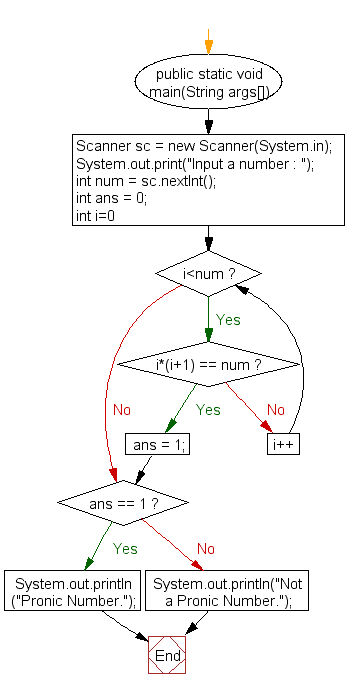﻿ Java exercises: Check whether a number is a Pronic Number or Heteromecic Number or not - w3resource# Java Exercises: Check whether a number is a Pronic Number or Heteromecic Number or not

## Java Numbers: Exercise-13 with Solution

Write a Java program to check whether a number is a Pronic Number or Heteromecic Number or not.

A pronic number is a number which is the product of two consecutive integers, that is, a number of the form n(n + 1).
The first few pronic numbers are:
0, 2, 6, 12, 20, 30, 42, 56, 72, 90, 110, 132, 156, 182, 210, 240, 272, 306, 342, 380, 420, 462 … etc.

Test Data
Input a number : 110

Pictorial Presentation:Sample Solution:

Java Code:

``````import java.util.Scanner;

public class Example13 {

public static void main(String args[])
{
Scanner sc = new Scanner(System.in);
System.out.print("Input a number : ");
int num = sc.nextInt();
int ans = 0;

for(int i=0; i<num; i++)
{
if(i*(i+1) == num)
{
ans = 1;
break;
}
}

if(ans == 1)
System.out.println("Pronic Number.");
else
System.out.println("Not a Pronic Number.");
}
}
```
```

Sample Output:

```Input a number : 110
Pronic Number.
```

Flowchart:Java Code Editor:

What is the difficulty level of this exercise?

﻿

## Java: Tips of the Day

Array vs ArrayLists:

The main difference between these two is that an Array is of fixed size so once you have created an Array you cannot change it but the ArrayList is not of fixed size. You can create instances of ArrayLists without specifying its size. So if you create such instances of an ArrayList without specifying its size Java will create an instance of an ArrayList of default size.

Once an ArrayList is full it re-sizes itself. In fact, an ArrayList is internally supported by an array. So when an ArrayList is resized it will slow down its performance a bit as the contents of the old Array must be copied to a new Array.

At the same time, it's compulsory to specify the size of an Array directly or indirectly while creating it. And also Arrays can store both primitives and objects while ArrayLists only can store objects.

Ref: https://bit.ly/3o8L2KH